# Design an Arduino Based Encoder Motor using PID Controller

Published  February 3, 2021   6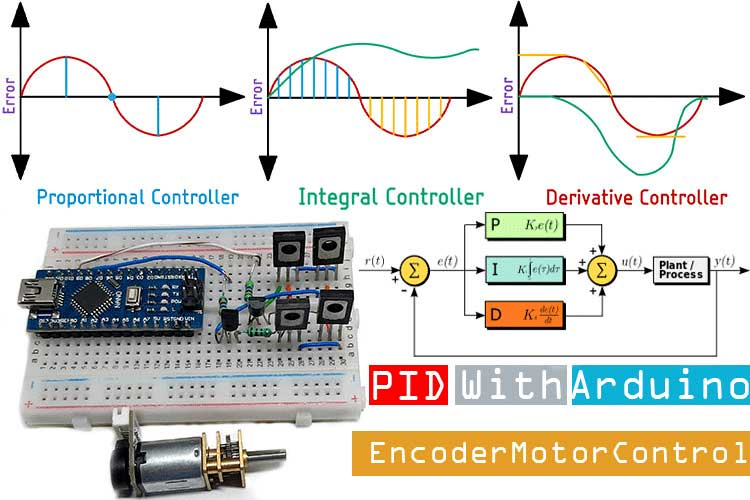In industrial automation and control, PID controller has become one of the most reliable control algorithms that can be implemented to stabilize the output response of any system. PID stands for Proportional-Integral-Derivative. These three types of control mechanism are so combined that it produces an error signal, and this error signal is used as feedback to control the end application. PID controllers can be found in a wide range of industrial and commercial applications like it's used to regulate pressure, linear movement, and many other variables. A PID temperature controller is the most common application that you can find on the internet. Without a PID controller, doing the job manually can be a tedious process. And in this era of advanced digital electronics and microcontrollers, it became easier to design and implement a PID controller in any system.

We decided to come up with an article about the PID controller, in which we will go into detail and understand its working principle. Next, we will take an example of our encoder motor and we will understand the problems that are associated with it. Finally, we will solve those problems by implementing a PID based control algorithm with our favourite microcontroller, Arduino.

In one of our previous articles, we have used PID control to make a self-balancing robot, you can check that out if you are interested in topics like that.

### What is a PID Controller and How does it Work?

As we have told you in the introduction section, PID is an acronym of proportional, integral, and derivative. But what does that even mean and is there any easier way to understand it? Yes, there is. For that, let’s take an example of DIY Smart Vacuum Cleaning Robot using Arduino which we did in one of our previous projects. To me, it’s a very cool project, and it’s very simple in terms of circuitry and control mechanism. But its major drawback is that it does not feature any PID-based control mechanism. Now, suppose the robot is cleaning itself and it comes near a staircase, it has a proximity sensor underneath the robot that detects such a situation and cuts the power to the motor, but because of inertia, the robot will not stop immediately. If that happens, the chances are high that the robot will trip down the staircase. Now, imagine you have a robocar, and you want to stop it in a certain position, this can be very difficult without PID because if you just cut the power, the car will absolutely miss its target because of its momentum. The image below will give you a better idea of the process.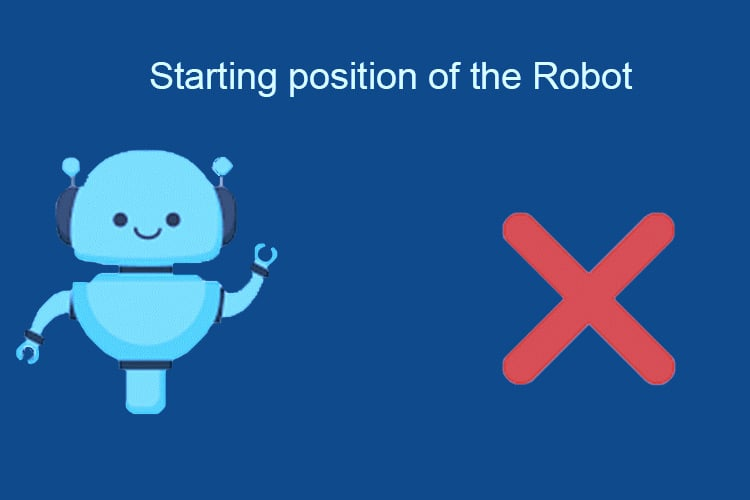Now as we know the concept, we can move forward and understand some of the advanced parts. If you search online for a PID controller, the first result you will get is from PID controller - Wikipedia, and in this post, you will find a block diagram along with an equation. But what this equation even means and how do we implement it in our microcontroller? Good question, now follow along and you will understand how,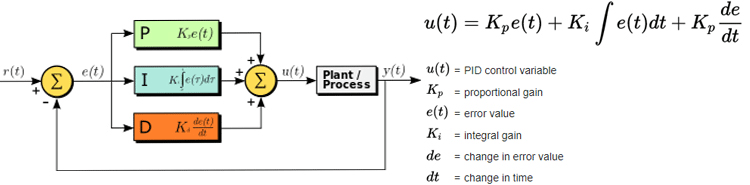This controller is named after, how an error is treated, before being summed, and then sent into the plant/process. Let me explain! In the block diagram, you can see that in the proportional path, the error is multiplied by a constant Kp. In the integral path, the error is multiplied by the constant Ki then it is integrated, and in the derivative path, it’s multiplied by Kd, and then differentiated. After that, the three values are summed together to produce the output. Now in the controller, the Kp, Kd and Ki, parameters are called the gain. And they are adjusted or tuned to fulfill a certain set of requirements and by changing these values, you can adjust how sensitive your system becomes to each of these different parameters, either the P, I, or D parameters. Let me explain it by individually examining each parameter.

The P-Controller: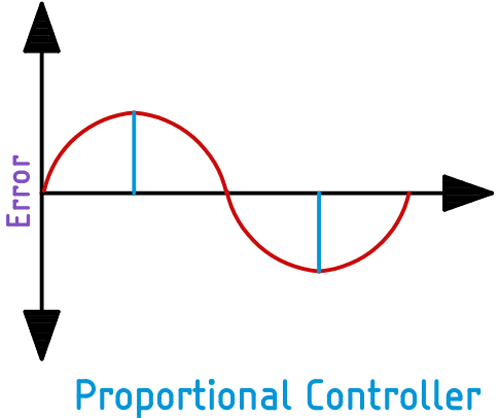Let’s say the error in the system is changing over time as you can observe in the red line. In a proportional controller, the output is the error that is defined by the gain Kp. As you can see, when the error is large, the output will produce a large output, and when the error is zero, the output error is zero and when the error is negative, the output is negative

The I-Controller: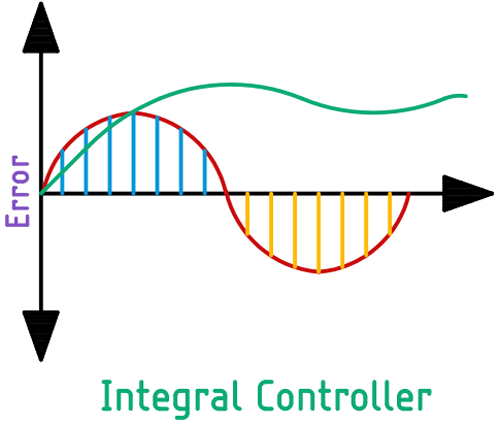In an integral controller, as the error value changes over time, the integral will start to sum up the error starts, and it will multiply it with constant Ki. In this type of controller, it’s easy to see that the integral result is the area underneath the curve where the area shown in blue is the positive area and the area shown in yellow is the negative area. In a complicated system, the integral controller is used to remove constant errors in a control system. No matter how small is the constant error, eventually, the summation of the errors will be enough to adjust the controller output. In the above picture, the error is represented with the green line.

The D-Controller: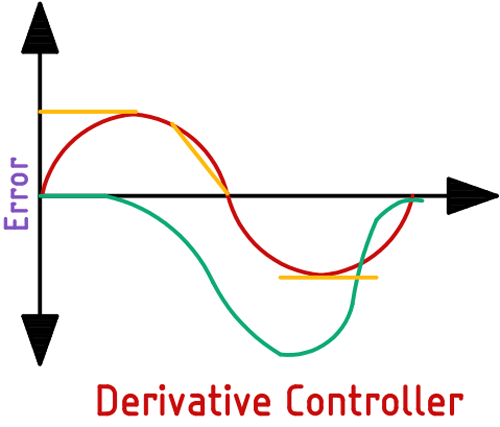In a derivative controller, it’s the rate of change of the error that contributes to the output signal. When the change in error is moving relatively slowly, as an example we can use the starting position of the sine wave. The derivative output will be small as you can see in the above picture (represented by the green line). And faster the error changes, the larger the output becomes.

Now at this point, you can sum up the three outputs and you have the PID controller. But often you do not need all three controllers working together, instead, we could remove anyone by setting the setpoint to zero. For example, we can have a PI controller by setting the D value to zero, else we can have a PD controller by setting the I parameter to zero. Now that we have a clear idea, we can move onto the actual hardware example.

### What is an Encoder Motor and how does it Work?

The concept of an encoder motor is very simple: It's a brushed DC motor that has an encode attached to it. In a previous article, we have talked all about rotary encoders in detail, you can check that out if you want to know more about the topic.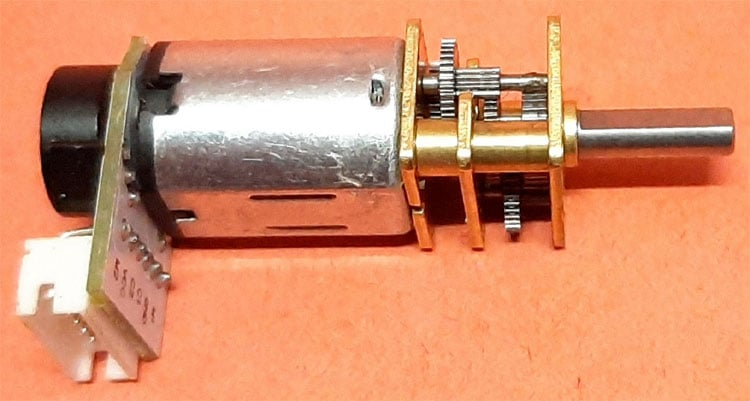In an encoder motor, a rotary encoder is mounted to a DC motor which provides feedback to the system by tracking the speed or the position of the motor shaft. There are many different types of motors available and all those can have different types of encoder configuration such as incremental or absolute, optical, hollow shafted, magnetic and the list goes on and on. Different types of motors are made for different types of applications. Not only DC motors but many servo motors, stepper motors, and AC motors come with a built-in encoder. In the above image, you can see an N20 permanent magnet type encode motor, which reduces the output RPM to 15 with the help of an attached gearbox. You can also see two hall sensors attached to the PCB. These hall sensors pick up the direction in which the motor is rotating, and with the help of a microcontroller, we can read it very easily.

### Components Required to Build a PID Enabled Encoder Motor Controller

At this point, we have a good idea about the working of a PID controller and we also know our end objective. Based on that we have decided to use an Arduino and some other complementary components to build a circuit. A list of those complementary components is shown below.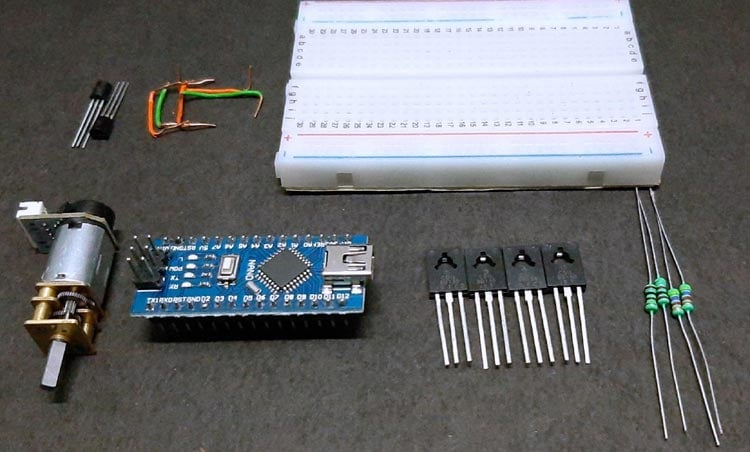• Arduino Nano - 1
• N20 Encode Motor - 1
• BD139 - 2
• BD140 - 2
• BC548 - 2
• 100R Resistor - 2
• 4.7K Resistor - 2
• Jumper Wires
• Power Supply

### Schematic Diagram for Testing PID Enabled Encoder Motor Controller

The complete Schematic Diagram for the PID Enabled Encoder Motor Controller is shown below. The working principle of this circuit is very simple, and it's described below.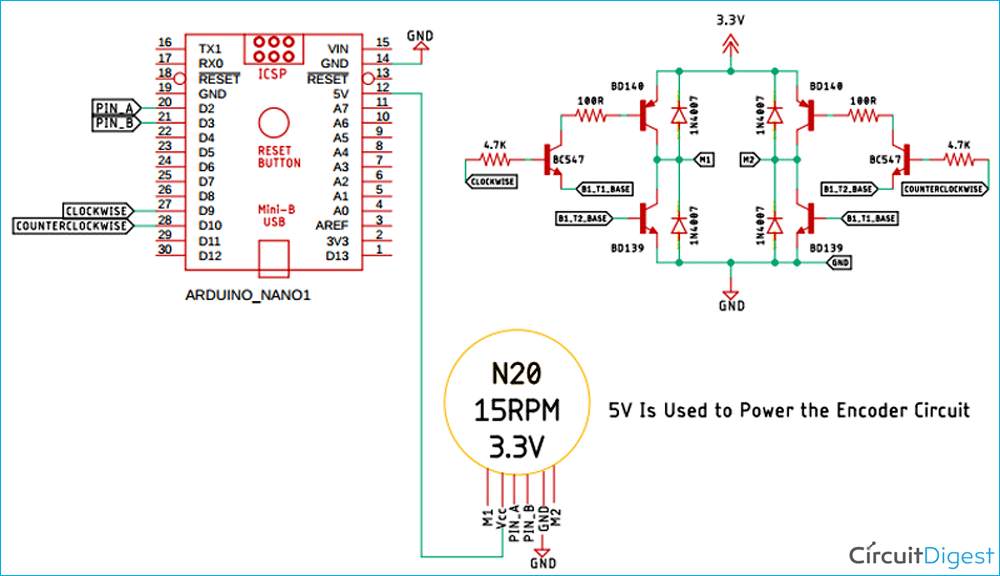The circuit is very simple. First, in the Schematic, we have the N20 Encoder motor which has Six Pins, the Pins are tagged as M1, M2 which are used to power the motor, as this is a very small motor rated at 3.3V. Next, we have the VCC and GND Pins which are used to power the encoder circuitry.  To power the encoder circuitry, you have to give it +5V otherwise, the encoder circuit will not work properly. Next, we have the PIN_A and PIN_B of the motor. These two pins are directly connected to the encoder. By reading the status of these pins, we can easily measure the RPM, this 15RPM N20 motor has a 1:2098 gear ratio which means the main motor shaft needs to rotate 2098 times for the auxiliary shaft to rotate once. The PIN_A and PIN_B are connected to the Pin 9 and pin 10 of the Arduino pin 9 and 10 are both PWM capable pins; the selected pins must have PWM functionality otherwise the code will not work. The PID controller controls the motor by controlling the PWM.

Next, we have our H-bridge motor driver, the motor driver is so made that we can control the motor by only using two pins of the Arduino and it even prevents the motor from false triggering.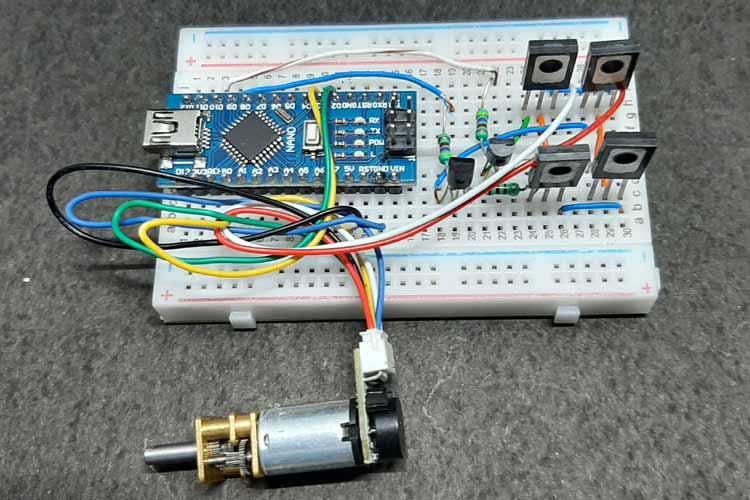### Arduino Code for PID Enabled Encoder Motor Controller

The complete code used in this project can be found at the bottom of this page. After adding the required header files and source files, you should be able to directly compile the Arduino code without any errors. You can download the PID controller library from the link given below or else you can use the board manager method to install the library.

The explanation of the code in the ino. File is as follows. First, we start by including all the required libraries. In this program, we are only using the PID controller library, so we need to include that first. After that, we define all the necessary pins that are required to read the encoder and drive the motor. Once that is done, we define all the values for the Kp, Ki, and Kd.

```#include <PIDController.h>
/* ENCODER_A and ENCODER_B pins are used to read the encoder
* Data from the microcontroller, the data from the encoder
* comes very fast so these two pins have to be interrupt enabled
* pins
*/
#define ENCODER_A 2
#define ENCODER_B 3
/* the MOTOR_CW and MOTOR_CCW pins are used to drive the H-bridge
* the H-bridge then drives the motors, These two pins must have to
* be PWM enabled, otherwise the code will not work.
*/
#define MOTOR_CW 9
#define MOTOR_CCW 10```

Next, we have defined the __Kp, __Ki, and __Kd values for our code. These three constants are responsible for setting up the output response for our code. At this point, please do note that for this project, I have used the trial-and-error method to set the constants, but there exist other methods that do the job very well.

```/*In this section we have defined the gain values for the
* proportional, integral, and derivative controller I have set
* the gain values with the help of trial and error methods.
*/
#define __Kp 260 // Proportional constant
#define __Ki 2.7 // Integral Constant
#define __Kd 2000 // Derivative Constant```

Next, we have defined all the necessary variables that are required in this code. First, we have the encoder_count variable that is used to count the number of interrupts that are generated; thus it counts the number of turns. Next, we have defined an unsigned int type variable integer value that stores the value which we have put in the serial monitor. Next, we have defined a char type variable incomingByte that temporarily stores the incoming serial data. Next, we have defined the most important variable in this code, and it's the motor_pwm_value variable after the data is computed through the PWM algorithm it's stored in this variable. When these variables are defined, we make an instance for the PID controller. Once we do this, we can move onto our setup() function.

```volatile long int encoder_count = 0; // stores the current encoder count
unsigned int integerValue = 0; // stores the incoming serial value. Max value is 65535
char incomingByte; // parses and stores each character one by one
int motor_pwm_value = 255; // after PID computation data is stored in this variable.
PIDController pid_controller;```

In the setup function, we have assigned the ENCODER_A and the ENCODER_B pins as input and we have defined MOTOR_CW and MOTOR_CCW pins as output. Next, we have assigned the ENCODER_A as an interrupt, and upon the RISING edge, this will call the function encoder(); next three lines are once again most important as we have enabled the PID controller with begin() method, and we also tuned the controller with the Kp, Ki and Kd values. And finally, we have set the limit for our PID controller output.

```void setup() {
Serial.begin(115200); // Serial for Debugging
pinMode(ENCODER_A, INPUT); // ENCODER_A as Input
pinMode(ENCODER_B, INPUT); // ENCODER_B as Input
pinMode(MOTOR_CW, OUTPUT); // MOTOR_CW as Output
pinMode(MOTOR_CCW, OUTPUT); // MOTOR_CW as Output
/* attach an interrupt to pin ENCODER_A of the Arduino, and when the pulse is in the RISING edge called the function encoder().
*/
attachInterrupt(digitalPinToInterrupt(ENCODER_A), encoder, RISING);
pidcontroller.begin(); // initialize the PID instance
pidcontroller.tune(__Kp , __Ki , __Kd); // Tune the PID, arguments: kP, kI, kD
pidcontroller.limit(-255, 255); // Limit the PID output between -255 to 255, this is important to get rid of integral windup!
}```

Next, we have our loop() section. In the loop section, we first check if the serial is available or not. If the serial is available, we parse the integer value and save it to the integer value variable. Next, we have a ‘/n’ character that is coming in. We put that in the incomingByte variable, and check this variable with an if statement, if true, we continue with the loop, next we set the target point with the pidcontroller.setpoint(integerValue); and pass on the integer value that we just received from serial. Next, we print the received value for debugging.

We have the motor_pwm_value variable and we compute the PID values and put it in this variable. If this value is greater than zero, we call the motor_ccw(motor_pwm_value) function and pass in the value, else, we call the motor_cw(abs(motor_pwm_value)) function. This marks the end of our loop section.

```void loop() {
while (Serial.available() > 0) {
integerValue = Serial.parseInt(); // stores the integerValue
incomingByte = Serial.read(); // stores the /n character
pidcontroller.setpoint(integerValue); // The "goal" the PID controller tries to "reach",
Serial.println(integerValue); // print the incoming value for debugging
if (incomingByte == '\n') // if we receive a newline character we will continue in the loop
continue;
}
motor_pwm_value = pidcontroller.compute(encoder_count);  //Let the PID compute the value, returns the calculated optimal output
Serial.print(motor_pwm_value); // print the calculated value for debugging
Serial.print("   ");
if (motor_pwm_value > 0) // if the motor_pwm_value is greater than zero we rotate the  motor in clockwise direction
MotorCounterClockwise(motor_pwm_value);
else // else, we move it in a counter-clockwise direction
MotorClockwise(abs(motor_pwm_value));
Serial.println(encoder_count);// print the final encoder count.
}
```

Next, we have the encoder function. This function is called when tan rising edge interrupts occurs in the ENCODER_B. When true, we check the statement again with if (digitalRead(ENCODER_B) == HIGH). Once true, we counter variable gets incremented. Otherwise, it gets decremented.

```void encoder() {
if (digitalRead(ENCODER_B) == HIGH) // if ENCODER_B is high increase the count
Encoder_count++; // increment the count
else // else decrease the count
Encoder_count--; // decrement the count
}
```

Next, we have the function which rotates the motor clockwise. When this function is called, it checks if the value is greater than 100 or not. If so, we rotate the motor in a clockwise direction otherwise we stop the motor.

```void motor_cw(int power) {
if (power > 100) {
analogWrite(MOTOR_CW, power);
digitalWrite(MOTOR_CCW, LOW);
}
// both of the pins are set to low
else {
digitalWrite(MOTOR_CW, LOW);
digitalWrite(MOTOR_CCW, LOW);
}
}
```

The same is true for the function which rotates the motor counter-clockwise. When this function is called, we check the value and rotate the motor counter-clockwise.

```void motor_ccw(int power) {
if (power > 100) {
analogWrite(MOTOR_CCW, power);
digitalWrite(MOTOR_CW, LOW);
}
else {
digitalWrite(MOTOR_CW, LOW);
digitalWrite(MOTOR_CCW, LOW);
}
}```

This marks the end of our coding section.

### Testing the PID Enabled Motor Controller

The following setup is used to test the circuit. As you can see, I have used an electrical box with some double-sided tape to hold the motor in place, and I have used a small buck converter module to power the motor as the motor runs on a 3.3V.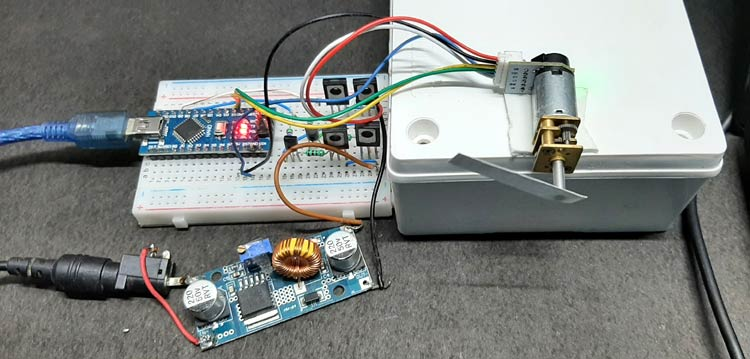As you can also see, we have connected a USB cable with the Arduino that is used to set the setpoint of the PID controller. We also get the debugging information from the Arduino with the USB. In this case, it gives the current encoder count. The image below will give you a better idea of the process.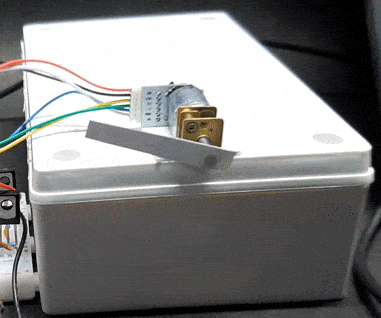This marks the end of the tutorial. I hope you liked the article and learned something new. If you have any questions regarding the article, you can leave them in the comment section below or you can use our Electronics Forum. You can also check out the video at the bottom of the page for better understanding.

Code
```#include <PIDController.h>
/* ENCODER_A and ENCODER_B pins are used to read the encoder
data from the microcontroller, the data from the encoder
comes very fast so these two pins have to be interrupt enabled
pins
*/
#define ENCODER_A 2
#define ENCODER_B 3
/* the MOTOR_CW and MOTOR_CCW pins are used to drive the H-bridge
the H-bridge then drives the motors, This two pins must have to
be PWM enabled, otherwise the code will not work.
*/
#define MOTOR_CW 9
#define MOTOR_CCW 10
/*In this section we have defined the gain values for the
proportional,integral, and derivative controller i have set
the gain values with the help of trial and error methods.
*/
#define __Kp 260 // Proportional constant
#define __Ki 2.7 // Integral Constant
#define __Kd 2000 // Derivative Constant
volatile long int encoder_count = 0; // stores the current encoder count
unsigned int integerValue = 0; // stores the incoming serial value. Max value is 65535
char incomingByte; // parses and stores each individual character one by one
int motor_pwm_value = 255; // after PID computation data is stored in this variable.
PIDController pidcontroller;
void setup() {
Serial.begin(115200); // Serial for Debugging
pinMode(ENCODER_A, INPUT); // ENCODER_A as Input
pinMode(ENCODER_B, INPUT); // ENCODER_B as Input
pinMode(MOTOR_CW, OUTPUT); // MOTOR_CW as Output
pinMode(MOTOR_CCW, OUTPUT); // MOTOR_CW as Output
/* attach an interrupt to pin ENCODER_A of the Arduino, and when the
pulse is in the RISING edge called the function encoder().
*/
attachInterrupt(digitalPinToInterrupt(ENCODER_A), encoder, RISING);
pidcontroller.begin(); // initialize the PID instance
pidcontroller.tune(260, 2.7, 2000); // Tune the PID, arguments: kP, kI, kD
pidcontroller.limit(-255, 255); // Limit the PID output between -255 to 255, this is important to get rid of integral windup!
}
void loop() {
while (Serial.available() > 0) {
integerValue = Serial.parseInt(); // stores the integerValue
incomingByte = Serial.read(); // stores the /n character
if (incomingByte == '\n') // if we receive a newline character we will continue in the loop
continue;
}
pidcontroller.setpoint(integerValue); // The "goal" the PID controller tries to "reach",
Serial.println(integerValue); // print the incoming value for debugging
motor_pwm_value = pidcontroller.compute(encoder_count);  //Let the PID compute the value, returns the calculated optimal output
Serial.print(motor_pwm_value); // print the calculated value for debugging
Serial.print("   ");
if (motor_pwm_value > 0) // if the motor_pwm_value is greater than zero we rotate the  motor in clockwise direction
motor_ccw(motor_pwm_value);
else // else we move it in a counter clockwise direction
motor_cw(abs(motor_pwm_value));
Serial.println(encoder_count);// print the final encoder count.
}
void encoder() {
if (digitalRead(ENCODER_B) == HIGH) // if ENCODER_B is high increase the count
encoder_count++; // increment the count
else // else decrease the count
encoder_count--;  // decrement the count
}
void motor_cw(int power) {
if (power > 100) {
analogWrite(MOTOR_CW, power); //rotate the motor if the value is grater than 100
digitalWrite(MOTOR_CCW, LOW); // make the other pin LOW
}
else {
// both of the pins are set to low
digitalWrite(MOTOR_CW, LOW);
digitalWrite(MOTOR_CCW, LOW);
}
}
void motor_ccw(int power) {
if (power > 100) {
analogWrite(MOTOR_CCW, power);
digitalWrite(MOTOR_CW, LOW);
}
else {
digitalWrite(MOTOR_CW, LOW);
digitalWrite(MOTOR_CCW, LOW);
}
}```
Video

Tags

Debashis, Thanks for the explanation, It’s as close to the closed loop stepper MKS42 that I have been able to find.  While I understand the theory, I get lost in the code.  In my project I am using Arduino Nano, ACS712 current sensors, and a MKS42 smart stepper driven through an DRV8825 expansion bd. Driver removed and adapter with cable to smart stepper.  I can run codes provided in GitHub and get expected results, but not finding code as above for that product.

My goal is to drive the stepper to a predetermined position (degree) when current sensor exceeds a set threshold triggered through analog port.  The detection part I have working.  Coding the smart stepper has got me stopped.  I can make a normal stepper go to a desired position but need the smart stepper, so it knows where it is.  If I can get a code to work for a single position, I can then code an array for the other sensor/positions.

Can you point me in the right direction or suggest a site I should go to?  Looking for one like you did but for the Smart stepper MKS, Bigtree,  MisFit,  etc.  Any help would be appreciated.

### Debashis,

Debashis,

The same circuit with a different set of codes may possibly create an indexing drive. Say 4 stops exactly at 90degree apart. Wait for 5 secs, then go to the next position. This may be 6 positions at 60 degree apart and stop for 10 secs and move to the next position. Can you try this?

Put a marker at each stop to check the accuracy of the location. Thanks

### sir kindly send me…

sir kindly send me simulation of this project and also send me the arduino nano1 library as well.i need this project urgently..plz help

### Hi, I like your write up, it…

Hi, I like your write up, it is a easy to follow description of the circuit. I did find a mistake and want point it out so other readers won't get confused. In the write-up PIN_A and PIN_B are said to connect to pins 9 &10 being PWM capable pins (output pins). They are actually connected to pins 2 & 3 and are inputs. The schematic is correct and I just wanted to point it out encase you wanted to correct the write-up.